# Structure and representation of organic molecules

Next, the structure and representation of organic molecules is discussed. First, a review of functional groups and the types of structural formulas to represent them is given. Subsequently, key concepts in Organic Chemistry such as electronegativity, bond polarity and hybridization are discussed. It continues with representations of Lewis structures and resonant ones. Finally, with a description of the most common types of representations for cyclic and acyclic organic compounds.

## Functional groups and structural formulas

Millions of organic compounds are described in the chemical literature, due to the almost unlimited capacity of carbon to form stable bonds with itself and with a wide range of elements.Carbon atoms can give carbon-carbon bonds by means of single, double or triple bonds, and can form chains and/or cycles, with the possibility of also having branches.

In addition, carbon can bind other elements such as H, O, N, S, halogens, Si, etc. so the possibilities are practically endless.
In the structure of an organic compound we can consider four levels:

a) Composition: indicates the atoms present in the compound and their relative proportion.
b) Constitution: indicates the connectivity of the different atoms in the compound. The main consequence is the concept of functional group, which is an atom or grouping of atoms that give a molecule certain properties.
c) Configuration: this tells us how the different atoms and functional groups are distributed in space. It would show us a three-dimensional image of the molecule.
d) Conformation: as a consequence of the more or less restricted rotation around the single bonds of the compounds. It would show us the relative arrangement around each of these bonds of the various substituents.

##• Empirical formula: the empirical formula describes the class of atoms in the molecule and the ratio of atoms present, e.g., -CH2 for ethene.
• Molecular formula: the molecular formula describes the exact number of atoms that make up a molecule. La fórmula molecular puede coincidir con la empírica o ser múltiplo de ésta, por ejemplo, C2H4 para el eteno.
• Fórmula estructural: the fórmula estructural describe el tipo de enlaces que hay en una molécula. La fórmula estructural puede ser:
• Fórmula desarrollada: en la fórmula desarrollada se representan todos los enlaces de la molécula. La fórmula estructuraldesarrollada es una representación gráfica que indica la manera de conectarse los átomos de una molécula determinada (los enlaces se muestran mediante líneas).
• Fórmula semidesarrollada: en la fórmula semidesarrollada,se suele simplificar la representación escribiendo los hidrógenos a continuación del átomo al que están unidos. Por ejemplo, CH3-CH2-CH3 para el propano.
• Fórmula simplificada: en la fórmula simplificada o esqueletal se representan las cadenas carbonadas y los ciclos mediante líneas, de forma que los átomos de carbono e hidrógeno se indican de una forma implícita. El extremo de una línea corresponde a un -CH3, la conjunción de dos líneas representa un -CH2, de tres un -CH y de cuatro un carbono cuaternario.

##• Number of unsaturations: information on the structure of the molecule can be obtained from the molecular formula, through the number of unsaturations. An unsaturation in a molecule may be due to the presence of:
• A double bond
• A cycle

Some common cases include:

• A triple bond provides two instaurations.
• The benzene ring provides four unsaturations (equivalent to three double bonds and one cycle).
• A pentagonal aromatic heterocycle is equivalent to three instaurations (two double bonds plus one cycle).

It is calculated using the following formula:• The expression does not include the number of oxygen atoms or other heteroatoms.
• If S atom appears in the formula it is not considered, although the number of instaurations can be distorted. There can be more instaurations but these will be on the sulfur atom.
• If halogens are present, they are considered equivalent to hydrogens.
• P (phosphorus) atom is considered equivalent to nitrogen, but we must make the same estimate as for sulfur.
• If Si atom is present, it is considered equivalent to carbon.
• Other heteroatoms are not considered.

### Hybridization and bonding

L.C. Pauling, in 1939, introduced the concept of orbital hybridization to satisfactorily explain the tetravalence of carbon and the geometry of organic molecules, although its electronic configuration is (1s2 2s2 2p2). According to this configuration, carbon should have valence 2, since the only electrons available would in principle be the two in the orbitals of the orbitals 2p. Thus, the geometry of, for example, of methane (CH4) is tetrahedral (109,47º) although the p orbitals form 90° angles between them.

The four valence orbitals (s y px, py, pz) of carbon combine to give 4 new hybrid orbitals sp3 as shown in the figure. The four new hybrid orbitals will adopt a geometrical arrangement that separates them as far as possible from each other, i.e., oriented according to the vertices of a tetrahedron (tetrahedrally).

##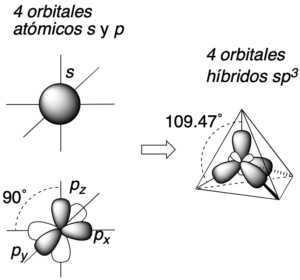In addition, when the carbon participates in multiple bonding or presents charge, other types of hybridizations such as sp2 o la sp, with trigonal-flat and linear geometry, respectively, can occur.

##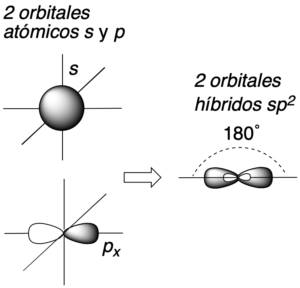When two carbon atoms are bonded together they can result in the formation of two types of bonds depending on their hybridization:

• σ bonds obtained by overlapping any two hybrid orbitals or between a hybrid orbital and an atomic orbital.
• π bonds obtained by overlapping two p orbitals that do not participate in the hybridization process.

Thus, a double bond will consist of one σ and one π bond and a triple bond will consist of one σ and two π bonds.

##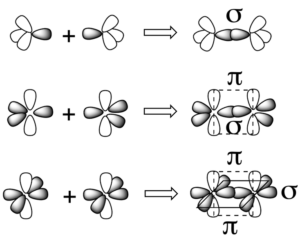It is necessary to indicate that the phenomenon of hybridization is not limited to the carbon atom, since other atoms such as O, N, P, and some more, possess similar hybridization types to carbon, although practically all atoms can undergo hybridization and it is due to the existence of a great diversity of possible geometries of chemical compounds, and not only organic. Thus, there is a direct relationship between the hybridization of the atoms and the geometry of the molecule.

## Bond polarity and electronegativity

The electronegativity is a concept introduced by Linus Pauling in 1932, which describes the ability of an atom to attract an electron (see Electronegativity Table).

 Atom Electronegativity (δEN) H 2,20 Li 0,98 Be 1,57 B 2,04 C 2,55 N 3,04 O 3,44 F 3,98 Si 1,90 P 2,19 S 2,58 Cl 3,16

Bond polarity can be estimated from the difference in electronegativities of the forming atoms. If this value is low, the bond is said to be non-polar (covalent); if the value is high, it corresponds to a polar (ionic) bond, and the electrons will be located in the bond near the most electronegative atom. The bond dipole moment is defined as the vector m whose magnitude is the result of multiplying the bond distance (d) by the difference between the partial charges Δq = (δ+ – δ-), thus: ( m = Δq · r ).

This vector has its origin in the positive charge and ends in the negative one. It is commonly expressed in non-SI units, Debye (1 Debye = 3,33564·10–30 C m). In addition, the molecular dipole moment μ is defined as the vector sum of the dipole moments of all the bonds present in a molecule. Since it is a vector, it is necessary to know the molecular geometry before adding them, in order to know how the bond dipole moments are oriented in space.

For example, the following figure shows three molecules (CO2, BF3 y CCl4) which, although they have polar bonds (the bond dipole moment is indicated by arrows with origin in the least electronegative atom), in all cases the molecular dipole moment resulting from the vectorial sum is zero due to molecular symmetry.

## Acyclic compounds representation

In order to facilitate the transmission of chemical information, a series of rules and conventions for the structure and representation of organic molecules are used. Also, the most usual ways to pass from one representation to another are shown.

### Skeleton or skeletal representation

It is an abbreviated system of representing molecular structure. Skeletal representations are widely used in organic chemistry because of their simplicity and ease of use even for complex molecules.
Carbon chains formed by single bonds are represented by broken lines where both carbon and hydrogen atoms are shown implicitly, i.e. they are considered included without representing them as such, so that the end of a line represents a CH3- a vertex in the broken line a -CH2- the union of three strokes a CH and the union of four lines a quaternary carbon.

Double and triple bonds are represented by two or three parallel lines, respectively, between the carbons forming these bonds.

Cyclic molecules are represented by geometric figures in the plane while aromatic rings are represented by geometric figures with alternating double bonds in the cycle or by a cycle with a circle inside it. Atoms other than carbon and hydrogen are represented by their chemical symbol.

##Abbreviations are often used to simplify the representation and writing of molecules (see functional groups).

### Cram representation (dash-wedge diagram)

The plane on which it is drawn is taken as a reference, using different types of strokes to obtain a three-dimensional perspective on paper.

##### Sawhorse projection

The molecule is represented around a bond that is drawn with an oblique line simulating a certain perspective. The substituents attached to the carbons that define this bond are drawn forming 120º angles. Thus, the sawhorse projection for ethane would be:

##3D structure Ethane (eclipsed) Ethane (staggered)

### Newman projection

The structure is represented along a bond between two atoms in such a way that the carbon farthest from the observer is drawn with a circle, while the nearest is shown as a dot. The remaining three bonds of each carbon are drawn on the plane forming 120º angles. It is a particularly useful representation for conformational analysis. In eclipsed type conformations, to facilitate the view of the groups, the carbons are slightly rotated, thus avoiding their collapse.

##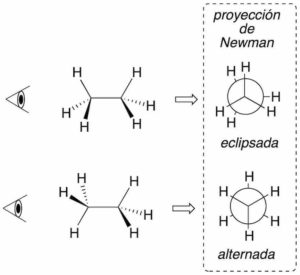### Fischer projection

This system is especially useful for the representation of carbon chains with one or more chiral carbons. A series of conventions are followed to perform this projection method:

• The carbon chain is drawn vertically.
• The atom with the highest priority locators is drawn toward the top.
• The groups drawn horizontally are placed so that they protrude outward from the plane in which the molecule is represented.

##Fischer projection allows easy detection of symmetry planes of the molecule, since it represents an eclipsed conformation. For example:

## Cyclic compounds representation

Cyclic compounds with 3, 4, 5 and 6 atoms are the most frequently studied in organic chemistry. We can find several methods of indicating the structure and representation of organic molecules.

### Regular polygons

Cyclic hydrocarbons are represented in the plane as regular polygons. The vertices indicate the carbon atoms. In addition, the substituents are represented by applying the same criteria as in the Cram representation.

##### Haworth projection

Frequently used in carbohydrate chemistry, it allows to describe the stereochemistry of cyclic compounds with convenience and to compare stereoisomers. The cycle is drawn on the plane with some perspective. In most cases this perspective is emphasized by using thick, wedge-shaped strokes.

Substituents are drawn using vertical lines. With this system of representation, meso forms are easily detected, since the planes of symmetry are intuitively located.

##### Perspective of confomers

Cyclopropane is planar, because the three carbons define only one plane, however, cyclobutane adopts a twisted conformation, while cyclopentane can present two types of conformations of similar energy called envelope and twisted.

##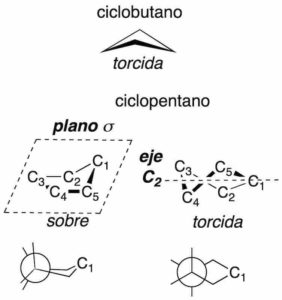The three most significant conformations of cyclohexane are as follows: a chair, a boat and a twisted boat. In the chair-type conformation if a hypothetical axis is drawn perpendicular to the cycle, the substituents parallel to that axis are called axial, while those lying obliquely are called equatorial. In addition, two adjacent substituents may be in relative cis or trans arrangement as described in the figure (black and white squares).

##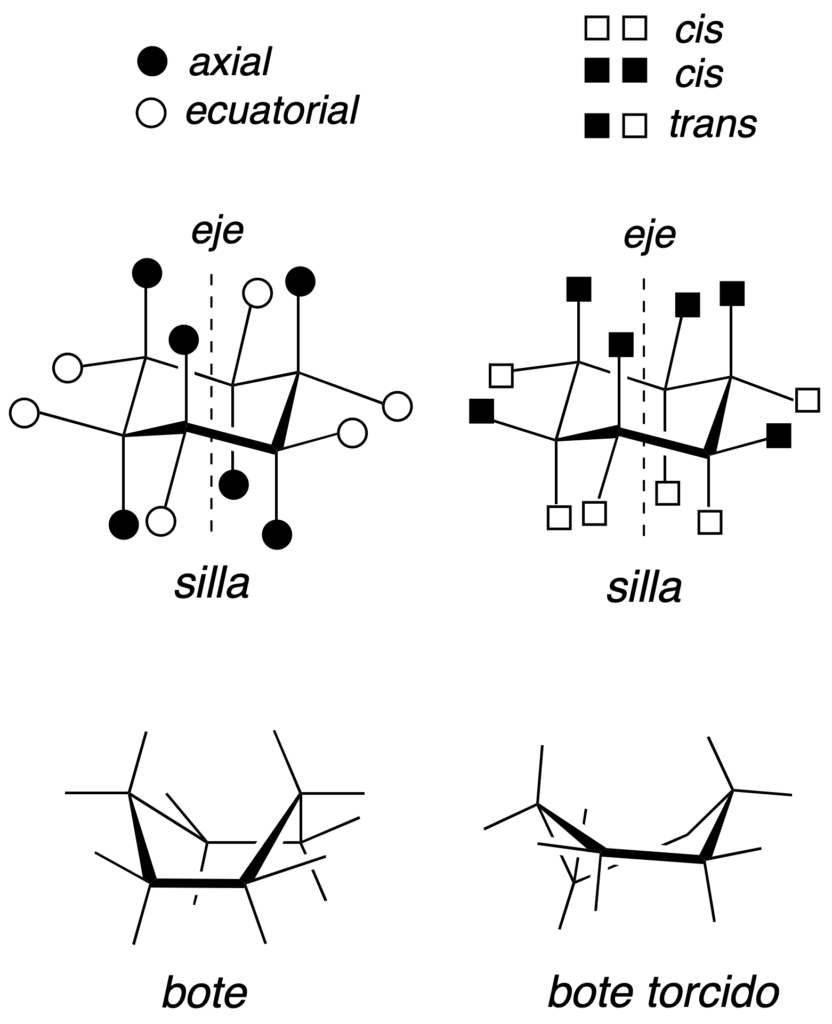### Newman projection

The most common ring in cycloalkanes is the cyclohexane, due to the importance of this structure. The hydrogens of carbons 1 and 4 are not usually represented, in order to simplify the drawing.## References and notes

 Le Bel and Van't Hoff were pioneers when they proposed in 1874 that the most probable orientation of the bonds in a carbon with 4 substituents occurs towards the vertices of a tetrahedron.

 The symbols δ+ and δ- indicate that there is positive and negative partial charge on each atom, respectively.